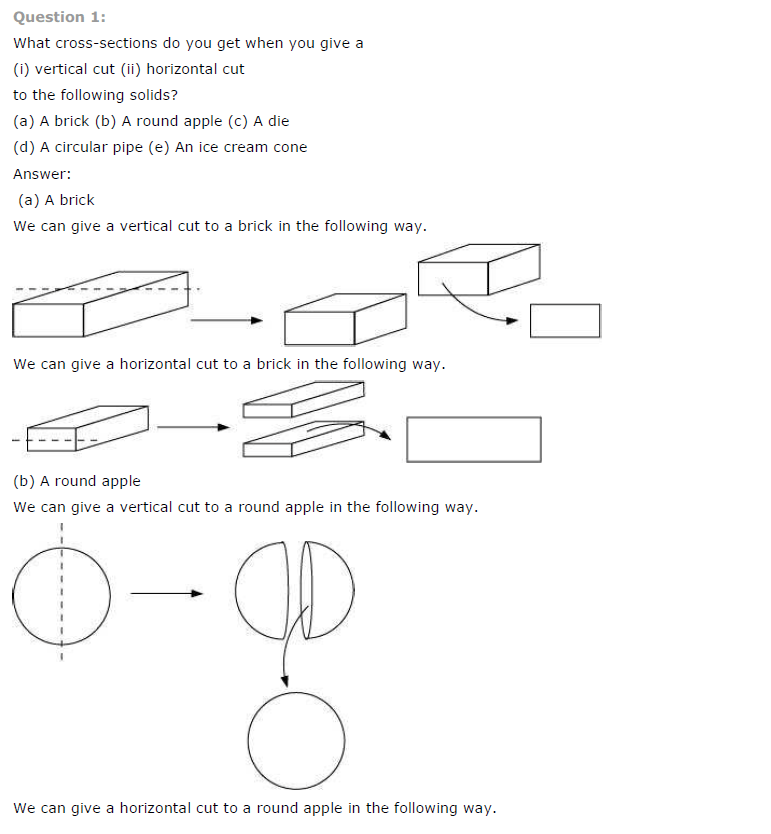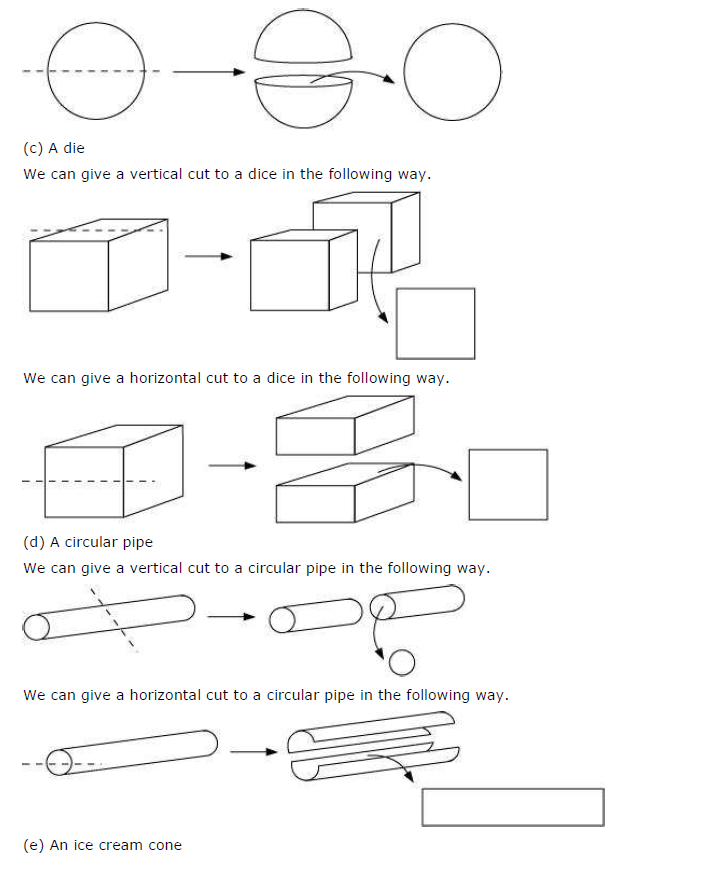# NCERT Solutions for Class 7 Maths Chapter 15 Visualising Solid Shapes Ex 15.3

NCERT Solutions for Class 7 Maths Chapter 15 Visualising Solid Shapes Ex 15.3

### NCERT Solutions for Class 7 Maths Chapter 15 Visualising Solid Shapes Ex 15.3

NCERT Solutions for Class 7 Maths Chapter 15 Visualising Solid Shapes Exercise 15.3
Ex 15.3 Class 7 Maths Question 1.
What cross-sections do you get when you give a
(i) vertical cut
(ii) horizontal cut to the following solids?
(a) A brick
(b) A round apple
(c) A die
(d) A circular pipe
(e) An ice cream cone.
Solution:

 Solids Shape of cross-section of vertical cut Shape of cross­section for horizontal cut (a) A brick Rectangle Rectangle (b) A round apple Circle Circle (c) A die Square Square (d) A circular pipe Circle Rectangle (e) An ice cream apple Triangle Circle## SabDekho

The Complete Educational Website